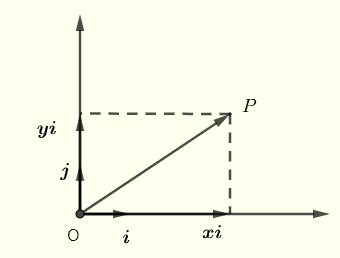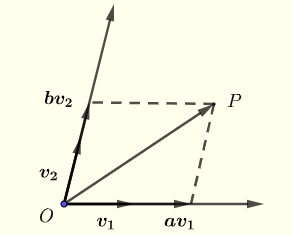# Basis, Coordinates and Dimension of Vector Spaces

Before we formally define the basis of a vector space, we give examples of basis in two dimensional space which you may already know from physics and/or analytical geometry which may help you understand the concept of basis.

## Definition of a Basis For 2-Dimensional Space Using Rectangular Axes

We first discuss what we know about vectors in a 2-dimensional space as used in physics and analytical geometry. The graph below shows point P , the orthogonal . unit vectors i and j and the vector OP .Vector OP may be expressed as a linear combination of the the unit vectors i and j such thatare called the coordinates of OP relative to the basis i,j.
   Using the parallelogram (which is here a rectangle), there is one way only to add two vectors $$x \textbf i$$ which is a multiple of vectors $$\textbf i$$ and $$y \textbf j$$ which is a multiple of vector $$\textbf j$$ to obtain vector $$\textbf{OP}$$
Take any vector in the plane of the coordinate system above and it can uniquely be represented as a sum of $$x \textbf i$$ and $$y \textbf j$$ as long as vector $$\textbf i$$ and $$y \textbf j$$ are not parallel (or linearly dependent).
Because we can express any vector in the 2-dimensional space using vectors $$\textbf i$$ and $$\textbf j$$, these two vectors form a basis for the 2-dimensional space.

## Generalize the Definition of a Basis For a 2-Dimensional Space

We now extend the above concept of basis using vectors that are not necessaritly orthogonal (see graph below). Vectors $$\textbf {v}_1$$ and $$\textbf {v}_2$$ do not have to be unit vectors or orthogonal.Using the parallelogram, there is is one way to add vectors $$a \textbf {v}_1$$ and $$b \textbf {v}_2$$ multiple of $$\textbf {v}_1$$ and $$\textbf {v}_2$$ respectively to obtain vector $$\textbf {OP}$$. In other words there is one way to write vector $$\textbf{OP}$$ as a linear combination of the basic vectors $$\textbf {v}_1$$ and $$\textbf {v}_2$$.
From the above, vector $$\textbf{OP}$$ may be written as a linear combination of any two vectors $$\textbf{v}_1$$ and $$\textbf {v}_2$$ in the 2-dimensional space as long as $$\textbf {v}_1$$ and $$\textbf {v}_2$$ are not parallel or linearly dependent. $\textbf{OP} = a \textbf {v}_1 + b \textbf {v}_2$ $$a$$ and $$b$$ are called the coordinates of $$\textbf {OP}$$ relative to the basis $${\textbf {v}_1 , \textbf {v}_2}$$.
Take any vector in the 2-dimensional space and it can uniquely be represented as a sum of $$a \textbf {v}_1$$ and $$b \textbf {v}_2$$ as long as vector $$\textbf {v}_1$$ and $$\textbf {v}_2$$ are not parallel (or linearly dependent).
Vectors $$v_1$$ and $$v_2$$ form a basis for the two dimensional space.

## Generalize the Definition of a Basis for a Subspace

We extend the above concept of basis of system of coordinates to define a basis for a vector space as follows:
If $$S = \{ \textbf {v}_1 ,\textbf {v}_2 , ... , \textbf {v}_n \}$$ is a set of vectors in a vector space $$V$$, then $$S$$ is called a basis for a subspace $$V$$ if
1)   the vectors in $$S$$ are linearly independent
2)  the vectors in $$S$$ span the subspace $$V$$

## Dimension of Vector Space

If $$S = \{ \textbf {v}_1 ,\textbf {v}_2 , ... , \textbf {v}_n \}$$ is a basis for vector $$V$$ then $$n$$, which is the number of vectors in the basis, is the dimension of the subspace $$V$$.

## Definition of Coordinates

We extend the above concepts of systems of coordinates using any basis for subspace as follows:
If $$S = \{ \textbf {v}_1 ,\textbf {v}_2 , ... , \textbf {v}_n \}$$ is a basis for vector $$V$$ and vector $$\textbf {u}$$ in $$V$$ may be written as a linear combination of the vectors in $$S$$ as follows
$\textbf {u} = r_1 \textbf {v}_1 + r_2 \textbf {v}_2 + ... + r_n \textbf {v}_n$
then the vector $$[\textbf {u}]_S = \begin{bmatrix} r_1 \\ r_2\\ . \\ . \\ r_n \end{bmatrix}$$ is called the coordinate vector of $$u$$ relative to the basis $$S$$.

## Examples with Solutions

Example 1
Is the set of vectors $$S = \left\{ \begin{bmatrix} -1 \\ 1 \\ 0 \\ \end {bmatrix} , \begin{bmatrix} 1 \\ 3 \\ -1 \\ \end {bmatrix} , \begin{bmatrix} -1 \\ 1 \\ 0 \\ \end {bmatrix} \right \}$$ a basis for a subspace of $$\mathbb{R}^3$$?

Solution to Example 1
We first need to find out if the vectors in the set $$S$$ are linearly independent by solving for $$r_1, r_2$$ and $$r_3$$ the equation
$$r_1 \begin{bmatrix} -1 \\ 1 \\ 0 \end {bmatrix} + r_2\begin{bmatrix} 1 \\ 3 \\ -1 \end {bmatrix} + r_3 \begin{bmatrix} -1 \\ 1 \\ 0 \end {bmatrix} = \begin{bmatrix} 0 \\ 0 \\ 0 \end {bmatrix}$$
The above equation in matrix form is given by
$$\begin{bmatrix} -1 &1&-1\\ 1 &3&1 \\ 0 &-1&0 \end{bmatrix} \begin{bmatrix} r_1 \\ r_2 \\ r_3 \end{bmatrix} = \begin{bmatrix} 0 \\ 0 \\ 0 \end {bmatrix}$$        (I)
The augmented matrix of the above homogeneous system is
$$\begin{bmatrix} -1 &1&-1&|&0\\ 1 &3&1&|&0 \\ 0 &-1&0&|&0 \end{bmatrix}$$
A homogeneous system of equation has either one solution only which is the trivial solution $$\begin{bmatrix} 0 \\ 0 \\ 0 \end {bmatrix}$$ and therefore the vectors in the set $$S$$ are linearly independent or an infinite number of solutions and the vectors in $$S$$ are linearly dependent .
Let us write the above augmented matrix in row echelon form.
Add row 1 and row 2 and put the result in row 2
$$\begin{bmatrix} -1 &1&-1&|&0\\ 0 &4&0&|&0 \\ 0 &-1&0&|&0 \end{bmatrix}$$
Add 4 times row 3 and row 2 and put the result in row 4
$$\begin{bmatrix} -1 &1&-1&|&0\\ 0 &4&0&|&0 \\ 0 &0&0&|&0 \end{bmatrix}$$
Multiply row 1 by -1 and row 2 by 1/4 to obtain
$$\begin{bmatrix} 1 & - 1 & 1&|&0\\ 0 &1&0&|&0 \\ 0 &0&0&|&0 \end{bmatrix}$$
The above matrix has pivots at columns 1 and 2 and therefore $$r_1$$ and $$r_2$$ are the basic variables. Solve in terms of $$r_3$$.
The last equation may be written as
$$0.r_3 = 0$$ , hence $$r_3$$ may take any real value.
The second equation gives
$$r_2 = 0$$
The first equation gives
$$r_1 = - r_3$$
The solution may be written as
$$\begin{bmatrix} r_1 \\ r_2 \\ r_3 \end{bmatrix} = \begin{bmatrix} -r_3 \\ 0 \\ r_3 \end{bmatrix}$$
As $$r_3$$ may take any real value, an infinite number of solutions, different from the trivial solution except the solution for $$r_3 = 0$$, may be generated and therefore the vectors in $$S$$ are linearly dependent which means that the set of vectors in $$S$$ is not a basis for $$\mathbb{R}^3$$.
NOTES
1) when the coefficient matrix is a square matrix as in the case of example 1, we could have used the determinant of the matrix. Using the last row of the square matrix in (I), the determinant is equal to zero and therefore the system has an infinite number of different solutions and only one of them is the trivial solution and therefore we could have reached the same conclusion as above.
2) A simpler way to test for linearity of vectors is included.

Example 2
Given the basis $$S = \left\{ \begin{bmatrix} 1 \\ 0 \\ 1 \\ 0 \end{bmatrix} , \begin{bmatrix} 0 \\ 2\\ 1\\ 0 \end{bmatrix} , \begin{bmatrix} 1 \\ 1\\ -1\\ 1 \end{bmatrix} \right \}$$ for a subspace $$V$$ and vector $$\textbf u = \begin{bmatrix} 4 \\ -3 \\ 0 \\ 1 \end{bmatrix}$$ in $$V$$.
a) What is the dimension of the subspace $$V$$?
b) Find the coordinates $$[\textbf u]_S$$ using the definition of coordinates given above.

Solution to Example 2
a)
The dimension of the subspace $$V$$ is given by the number of vectors in its basis; hence it is equal to $$3$$.

b)
Using the definition above, the coordinates $$[\textbf u]_S$$ of vector $$\textbf {u}$$ in basis $$S$$ are the constant $$r_1, r_2 , r_3$$ such that
$\begin{bmatrix} 4 \\ -3 \\ 0 \\ 1 \end{bmatrix} = r_1 \begin{bmatrix} 1 \\ 0 \\ 1 \\ 0 \end{bmatrix} + r_2 \begin{bmatrix} 0 \\ 2\\ 1\\ 0 \end{bmatrix} + r_3 \begin{bmatrix} 1 \\ 1\\ -1\\ 1 \end{bmatrix}$
The above system of equations in augmented matrix form is written as follows
$$\begin{bmatrix} 1 & 0 & 1 &|& 4\\ 0 & 2 & 1 &|& -3 \\ 1 & 1 & -1 &|& 0 \\ 0 & 0 & 1 &|& 1 \end{bmatrix}$$
Row reduce the above agmented matrix
$$\begin{bmatrix} 1 & 0 & 0 &|& 3\\ 0 & 1 & 0 &|& -2 \\ 0 & 0 & 1 &|& 1 \\ 0 & 0 & 0 &|& 0 \end{bmatrix}$$
The above matrix in row reduced form gives the solutions: $$r_1 = 3, r_2 = -2 , r_3 = 1$$
hence
$$[\textbf u]_S = \begin{bmatrix} 3\\ -2 \\ 1 \\ \end{bmatrix}$$

Example 3
Given the basis $$S_1 = \left\{ \begin{bmatrix} 1 \\ 2 \end{bmatrix} , \begin{bmatrix} -3 \\ 1 \end{bmatrix} \right\}$$ and $$[\textbf u]_{S_1} = \begin{bmatrix} -1 \\ 2 \end{bmatrix}$$ , find $$[\textbf u]_{S_2}$$ given the basis $$S_2 = \left\{ \begin{bmatrix} 0 \\ -1 \end{bmatrix} , \begin{bmatrix} -1 \\ 2 \end{bmatrix} \right\}$$ .

Solution to Example 3
Given $$[\textbf u]_{S_1}$$, $$\textbf u$$ is given by
$$\textbf u = -1 \begin{bmatrix} 1 \\ 2 \end{bmatrix} + 2 \begin{bmatrix} -3 \\ 1 \end{bmatrix} = \begin{bmatrix} -7 \\ 0 \end{bmatrix}$$
Knowing $$\textbf u$$, we now calculate $$[\textbf u]_{S_2} = \begin{bmatrix} r_1 \\ r_2 \end{bmatrix}$$ using the definition of the coordinates given above.
$$\begin{bmatrix} -7 \\ 0 \end{bmatrix} = r_1 \begin{bmatrix} 0 \\ -1 \end{bmatrix} + r_2 \begin{bmatrix} -1 \\ 2 \end{bmatrix}$$
Write the above system in augmnented matrix form
$$\begin{bmatrix} 0 & -1 & | & -7\\ -1 & 2 & | & 0 \end{bmatrix}$$
Row reduce
$$\begin{bmatrix} 1 & - 2 & | & 0\\ 0 & 1 & | & 7 \end{bmatrix}$$
Solve
$$r_2 = 7$$ and $$r_1 =14$$
$$[\textbf u]_{S_2} = \begin{bmatrix} 14 \\ 7 \end{bmatrix}$$

Example 4
Find a basis for the subspace of $$R^3$$ spanned by the vectors
$$\begin{bmatrix} 1 \\ 1 \\ 2 \end{bmatrix}$$ , $$\begin{bmatrix} -1 \\ -2 \\ -7 \end{bmatrix}$$ , $$\begin{bmatrix} 1 \\ 0 \\ -3 \end{bmatrix}$$ , $$\begin{bmatrix} 2 \\ 0 \\ 6 \end{bmatrix}$$

Solution to Example 4
Start by creating a matrix whose columns are the given vector as follows
$$\begin{bmatrix} 1 & -1 & 1 & 2\\ 1 & -2 & 0 & 0 \\ 2 & -7 & -3 & 6 \end{bmatrix}$$
Write the matrix in row reduced form
$$\begin{bmatrix} 1 & 0 & 2 & 0\\ 0 & 1 & 1 & 0 \\ 0 & 0 & 0 & 1 \end{bmatrix}$$
Columns 1, 2 and 4 have a pivot and therefore the original columns vectors $$\begin{bmatrix} 1 \\ 1 \\ 2 \end{bmatrix}$$ , $$\begin{bmatrix} -1 \\ -2 \\ -7 \end{bmatrix}$$ , $$\begin{bmatrix} 2 \\ 0 \\ 6 \end{bmatrix}$$ corresponding to these columns form a basis for the given subspace.